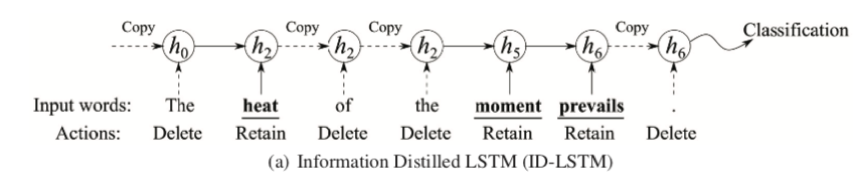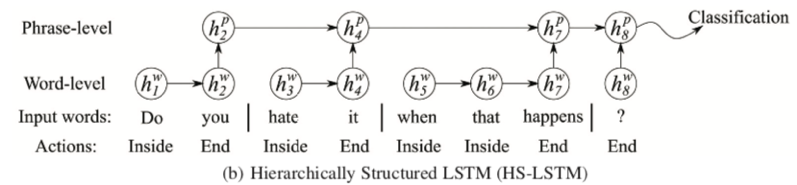# 一种强化学习在NLP文本分类上的应用模型

Information Distilled LSTM (ID-LSTM)

if $\alpha_t=retain$ then $c_t,h_t=LSTM(c_{t-1},h_{t-1},x_t)$
if $\alpha_t=delete$ then $c_t,h_t=c_{t-1},h_{t-1}$Hierarchically Structured LSTM (HS-LSTM)

if $\alpha_t=End$ then $c_t^w,h_t^w=\phi^w (0,0,x_t)$
if $\alpha_t=Inside$ then $c_t^w,h_t^w=\phi^w (c_{t-1}^w,h_{t-1}^w,x_t)$

if $\alpha_t=End$ then $c_t^p,h_t^p=\phi^p(c_{t-1}^p,h_{t-1}^p,h_t^w)$
if $\alpha_t=Inside$ then $c_t^p,h_t^p=c_t^{p-1},h_t^{p-1}$CNet对应的分类公式为$P(y|X)=softmax(W_s*h_L^p+b_s)$

1 预训练CNet网络和分类网络参数；对于ID-LSTM直接使用原始的不经过删减的句子进行预训练；对于HD-LSTM则先使用简单的启发式算法对原始句子中的word进行划分phrase处理；
2 固定住CNet部分网络参数，对PNet网络参数进行预训练操作；
3 Jointly 训练整个网络参数。

©️2019 CSDN 皮肤主题: 编程工作室 设计师: CSDN官方博客